## Weighted Job Scheduling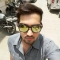Updated on 17-Jun-2020 07:53:36
A list of different jobs is given, with the starting time, the ending time and profit of that job are also provided for those jobs. Our task is to find a subset of jobs, where the profit is maximum and no jobs are overlapping each other.In this algorithm, we use a table to store the results of sub-problems and using the results of subproblems, the whole problem can be solved in a bottom-up manner.The time complexity of this algorithm is O(n^2), but we can change it to O(n Log n) by using a binary search method to search con-conflicting jobs.Input ... Read More

## Ugly Numbers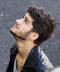Updated on 17-Jun-2020 08:04:29
Ugly numbers are those number whose prime factors are 2, 3 or 5. From 1 to 15, there are 11 ugly numbers 1, 2, 3, 4, 5, 6, 8, 9, 10, 12, 15. The numbers 7, 11, 13 are not ugly because they are prime. The number 14 is not ugly because in its prime factor the 7 will come.In this program, we will try to find the nth ugly number.Input and OutputInput: Take the term number. Say it is 10 Output: The 10th ugly number is 12AlgorithmgetUglyNumbers(n)Input: The number of terms.Output: Find nth Ugly numbers.Begin    define array named ... Read More

## Vertex cover Problem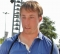Updated on 17-Jun-2020 08:06:13
For an undirected graph, the vertex cover is a subset of the vertices, where for every edge (u, v) of the graph either u or v is in the set.Using a binary tree, we can easily solve the vertex cover problem.This problem can be divided into two sub-problems. When the root is part of the vertex cover. For this case, the root covers all children edges. We can simply find the size of vertex cover for left and right sub-tree, and add 1 for the root.Input and OutputInput: A binary tree. Output: The vertex cover is 3.  AlgorithmvertexCover(root node)In this ... Read More

## Total number of non-decreasing numbers with n digits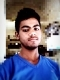Updated on 17-Jun-2020 08:08:33
A number is said to be non-decreasing when all digits (except the first place) is not smaller than its previous digit. For this algorithm, we have to find how many nondecreasing numbers are there in an N-digit number.Let count(n, d) a function to count how many non-decreasing numbers are there of length n and ending with letter d, then we can write the relation like this.$$count(n, d)=\displaystyle\sum\limits_{i=0}^d count(n-1, i)\total=\displaystyle\sum\limits_{d=0}^{n-1} count(n-1, d)$$Input and OutputInput: Number of digits, say 3. Output: The possible non decreasing numbers. Here it is 220. Non decreasing numbers are like 111, 112, 123, 789, 569 etc.AlgorithmcountNumbers(n)Input: The given ... Read More

## Shortest Common Super Sequence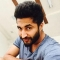Updated on 17-Jun-2020 08:09:32
Shortest common super-sequence is a sequence where each element of both of the given sequences is present. In other words, we can say that the given two strings, both are sub-sequence of Shortest Common Super-Sequence.When there are no common characters in two strings, then we can simply concatenate them to get Super-sequence. But when they have some common characters, then firstly we have to find the longest string, then add extra characters of the other string.Input and OutputInput: Two strings. “ABCDEF” and “XYDEF” Output: The length of the shortest common super-sequence. Here the super-sequence is “ABCDEFXY”. So the length is ... Read More

## Rod CuttingUpdated on 17-Jun-2020 08:11:06
A rod is given of length n. Another table is also provided, which contains different size and price for each size. Determine the maximum price by cutting the rod and selling them in the market.To get the best price by making a cut at different positions and comparing the prices after cutting the rod.Let the f(n) will return the max possible price after cutting a row with length n. We can simply write the function f(n) like this.f(n) := maximum value from price[i]+f(n – i – 1), where i is in range 0 to (n – 1).Input and OutputInput:The price ... Read More

## Partition problemUpdated on 17-Jun-2020 07:25:03
For this problem, a given set can be partitioned in such a way, that sum of each subset is equal.At first, we have to find the sum of the given set. If it is even, then there is a chance to divide it into two sets. Otherwise, it cannot be divided.For even value of the sum, then we will create a table named partTable, now use the following condition to solve the problem.partTable[i, j] is true, when subset of array to array[j-1] has sum equal to i, otherwise it is false.Input and OutputInput: A set of integers. {3, 1, 1, ... Read More

## Palindrome PartitioningUpdated on 17-Jun-2020 07:22:59
In this algorithm, the input is a string, a partitioning of that string is palindrome partitioning when every substring of the partition is a palindrome.In this algorithm, we have to find the minimum cuts are needed to palindrome partitioning the given string.Input and OutputInput: A string. Say “ababbbabbababa” Output: Minimum cut to partition as palindrome. Here 3 cuts are needed. The palindromes are: a | babbbab | b | ababaAlgorithmminPalPart(str)Input: The given string.Output: Minimum number of palindromic partitioning from the string.Begin    n := length of str    define cut matrix and pal matrix each of order n x n ... Read More

## Friend Pairing ProblemUpdated on 17-Jun-2020 07:29:15
In a group, there is n number of friends. Each person can remain single or be paired with some other friend. Find the total number of ways, in which friends can remain single or can be paired up.If one pair has two friends’ p and q, then (p, q) or (q, p) are same. For a group of n friends, let f(n) be the number of ways how they can be paired up or remain single. Then either the nth person remains single, or paired up. If the nth person is single, then we recur for (n - 1) friends. If ... Read More

## Wildcard Pattern MatchingUpdated on 17-Jun-2020 07:27:53
For this problem, one main string and another wildcard patterns are given. In this algorithm, it will check whether the wildcard pattern is matching with the main text or not.The wildcard pattern may contain letters or ‘*’ or ‘?’ Symbols. The ‘?’ Is used to match a single character and ‘*’ is used to match the sequence of characters including empty space.When the character is ‘*’: We can ignore the star character and move to check next characters in the pattern.When the next character is ‘?’, then we can ignore only the current character in the text, and check for ... Read More